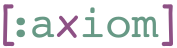## 1    symbols: Extract symbols from expressions

The `symbols` function is the key in our static analysis. It takes a s-expression, and returns a set of symbols defined by this s-expression.

For Constants, it returns an empty set.

``````(symbols 2) => #{}
(symbols true) => #{}
(symbols "foo") => #{}
(symbols :foo) => #{}
(symbols nil) => #{}
(symbols #"foobar") => #{}
(symbols *ns*) => #{}``````

For a symbol, `symbols` returns a set with that symbol.

``(symbols 'x) => #{'x}``

`symbols` goes into Clojure structures and aggergates symbols from there.

``````(symbols '(+ a b)) => #{'+ 'a 'b}
(symbols '[1 a 2 b]) => #{'a 'b}
(symbols {'x 'y}) => #{'x 'y}
(symbols #{'a 'b 3 4 5}) => #{'a 'b}``````

In a `let*` special form, the bindings are removed from the returned set.

``````(symbols '(let* [x 1 y 2] (+ x y))) => #{'+}
(symbols '(let* [x a] x)) => #{'a}``````

`symbols` expands macros, so it also handles the more familiar `let` form

``(symbols '(let [x a] x a)) => #{'a}``

`loop` is supported similarly.

``(symbols '(loop [x a y b] a x b y c)) => #{'a 'b 'c}``

In the `fn*` special form (used by the `fn` macro) `symbols` removes the argument names from the set.

``````(symbols '(fn [x y] (+ x y a))) => #{'a '+}
(symbols '(fn foo [x y]  (+ x (foo y a)))) => #{'a '+}``````

The function name (in named functions) is also removed.

``(symbols '(fn foo [x] (foo x))) => #{}``

Multi-arity functions are supported as well.

``````(symbols '(fn ([x] (+ a x))
([x y] (+ b x y)))) => #{'a 'b '+}``````

`def` is not allowed inside an expression (it is allowed on the top-level, as described below).

``(symbols '(def x 2)) => (throws "def is not allowed")``

Similarly, reference to variables is not allowed.

``(symbols '#'var) => (throws "vars are not allowed")``

Quoted symbols are ignored.

``(symbols ''(a b c)) => #{}``

In special forms such as `if` and `do`, the form's name is ignored.

``````(symbols '(if a b c)) => #{'a 'b 'c}
(symbols '(do a b c)) => #{'a 'b 'c}
(symbols '(recur a b)) => #{'a 'b}``````

Exceptions are not supported because they use Java interop.

``````(symbols '(throw foo)) => (throws Exception "throw is not allowed. Use error instead")
(symbols '(try foo bar baz)) => (throws "try/catch is not allowed")``````

While `for` is a macro and not a special form, its definition makes use of Java interop, which we disallow. We therefore make `for` a special case.

``(symbols '(for [x foo] (* x 2))) => #{'foo '*}``

Reader macros annonimous functions are supported.

``````(symbols '#(+ 1 %)) => #{'+}
(symbols '(fn* [x] (+ 1 x))) => #{'+}``````